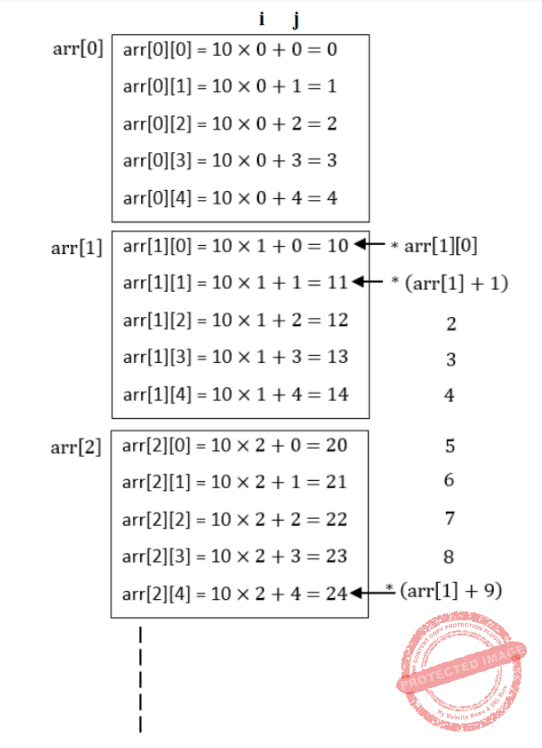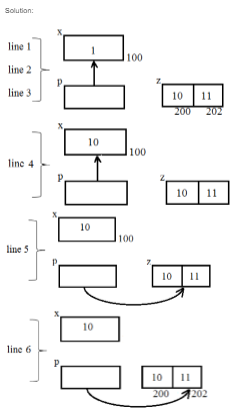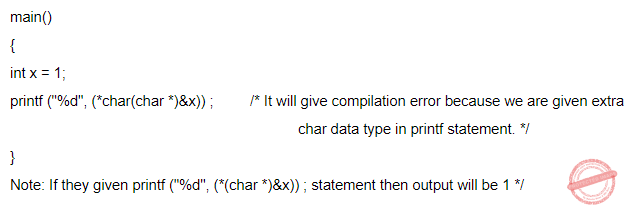## Pointers

 Question 1

Consider the following three C functions:

```[PI]            int*g(void)
{
int x = 10;
return(&x);
}

[P2]            int*g(void)
{
int*px;
*px = 10;
return px;
}

[P3]            int*g(void)
{
int*px;
px = (int *) malloc(sizeof(int));
*px = 10;
return px;
} ```

Which of the above three functions are likely to cause problems with pointers?

 A Only P3 B Only P1 and P3 C Only P1 and P2 D P1, P2 and P3
Data-Structures       Pointers       GATE 2001
Question 1 Explanation:
[P1] → May cause error because the function is returning the address of locally declared variable.
[P2] → It will cause problem because px is in int pointer that is not assigned with any address and we are doing dereferencing.
[P3] → It will work because memory will be stored in px that can be use further. Once function execution completes this will exist in Heap.
 Question 2

Faster access to non-local variables is achieved using an array of pointers to activation records called a

 A stack B heap C display D activation tree
Data-Structures       Pointers       GATE 1998
Question 2 Explanation:
Properties of displays:
→ Use a pointer array to store the activation records along the static chain.
→ Fast access for non-local variables but may be complicated to maintain.
 Question 3
Consider the following ANSI C program
#include<stdio.h>
int main() {
int arr;
int i, j;
for (i=0; i<4; i++){
for (j=0; j<5; j++){
arr[i][j] = 10*i + j;
}
}
printf (“%d”, *(arr + 9));
return 0;
}
What is the output of the above program?
 A 14 B 30 C 24 D 20
Programming       Pointers       GATE 2021 CS-Set-2       Video-Explanation
Question 3 Explanation:
arrQuestion 4
What is printed by the following ANSI C program?
#include
int main(int argc, char *argv[])
{
int x = 1, z = {10, 11};
int *p = NULL;
p = &x;
*p = 10;
p = &z;
*(&z + 1) += 3;
printf("%d, %d, %d\n", x, z, z);
return 0;
}
 A 1, 10, 11 B 1, 10, 14 C 10, 14, 11 D 10, 10, 14
C-Programming       Pointers       GATE 2022       Video-Explanation
Question 4 Explanation:Question 5
Consider the following programAssuming required header files are included and if the machine in which this program is executed is little-endian, then the output will be
 A 0 B 99999999 C 1 D unpredictable
Programming-for-Output-Problems       Pointers       ISRO-2018       Video-Explanation
Question 5 Explanation:There are 5 questions to complete.

Register Now